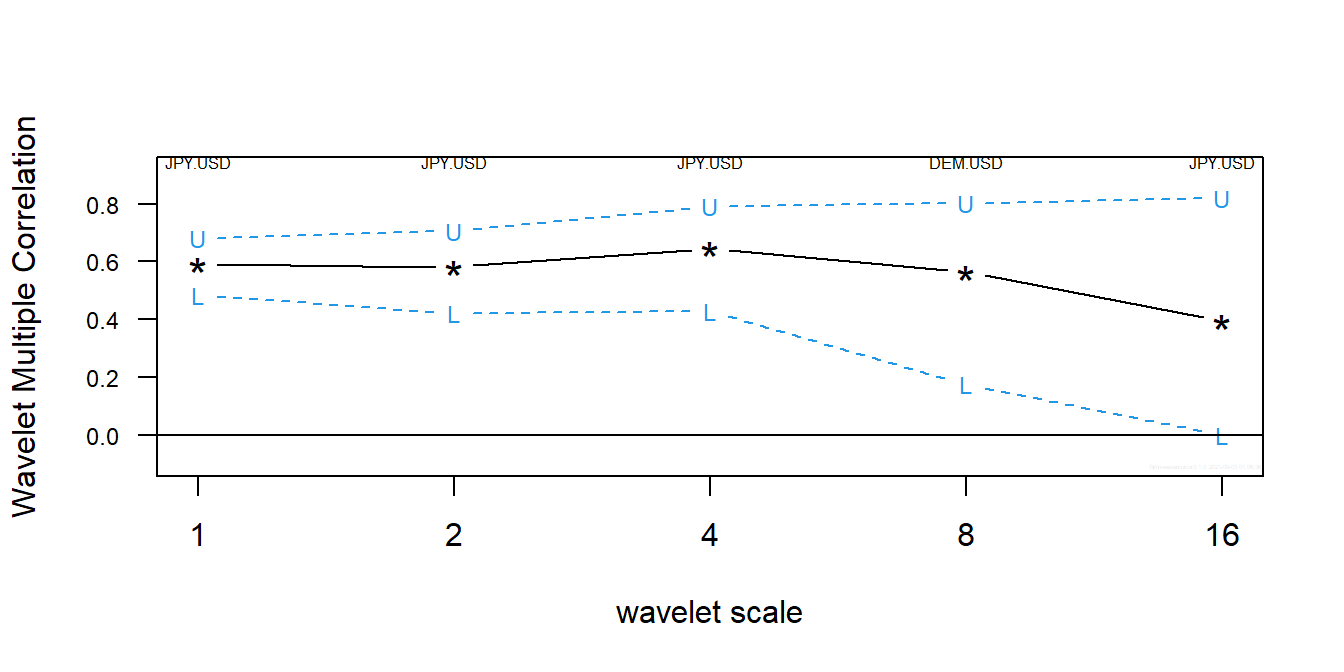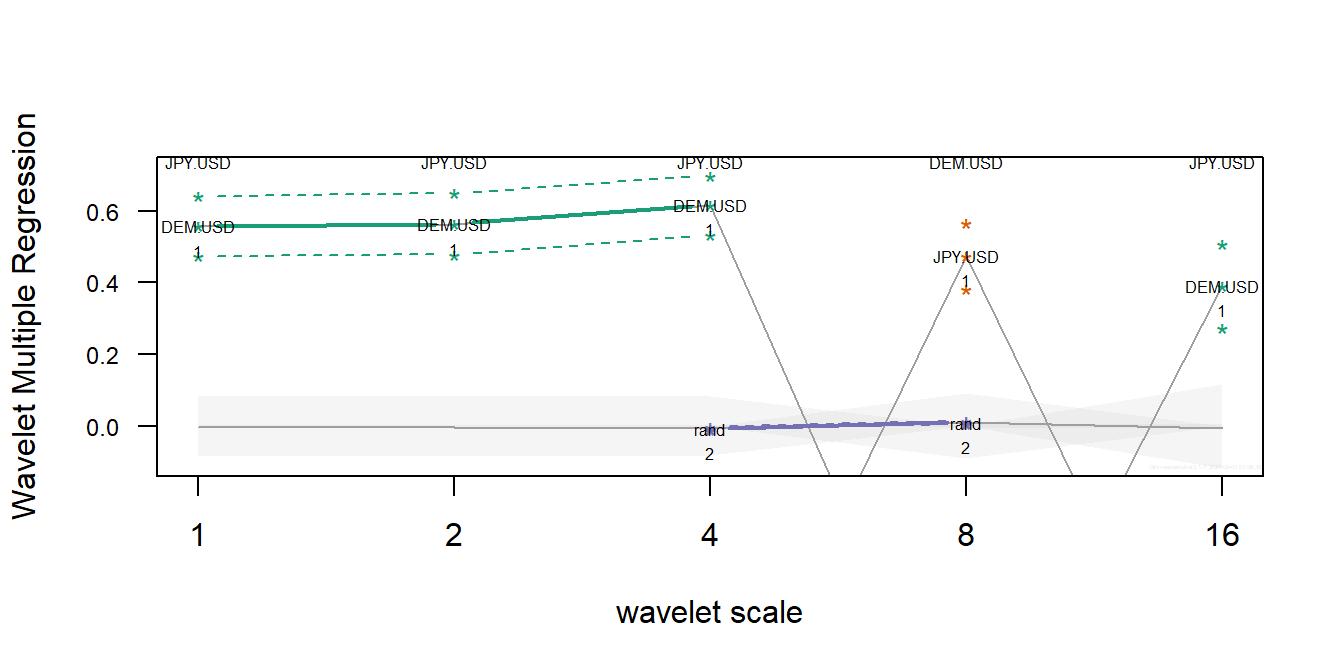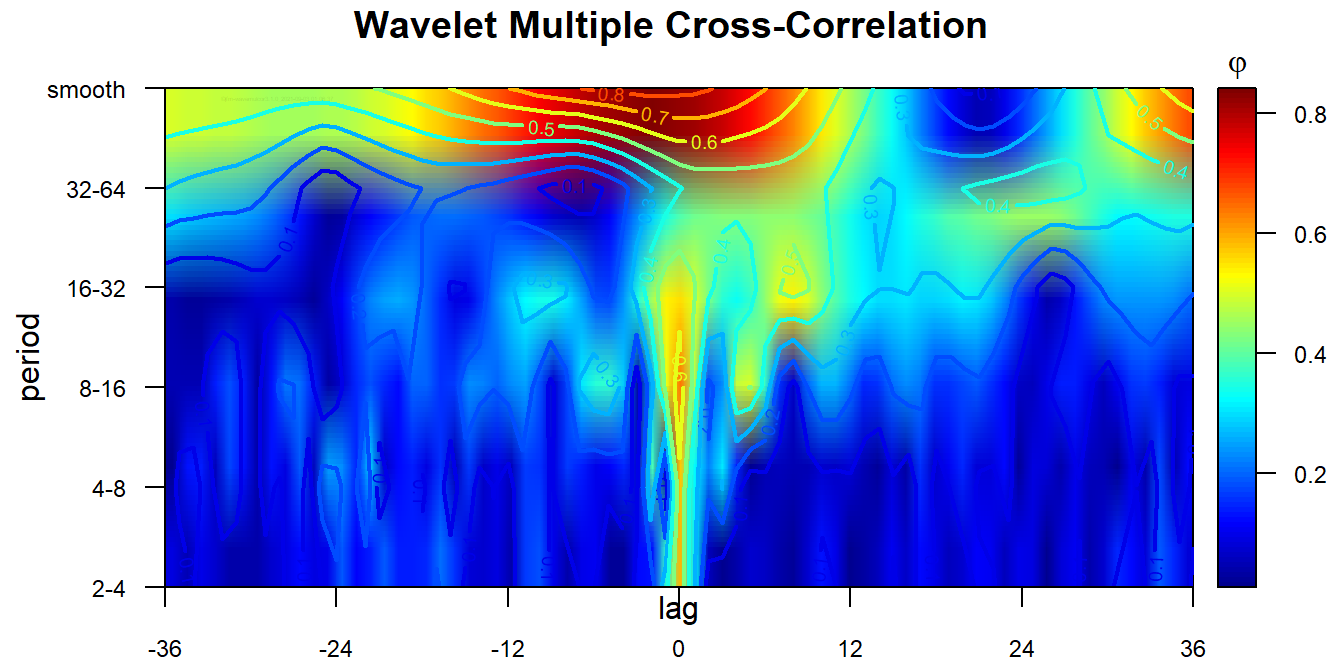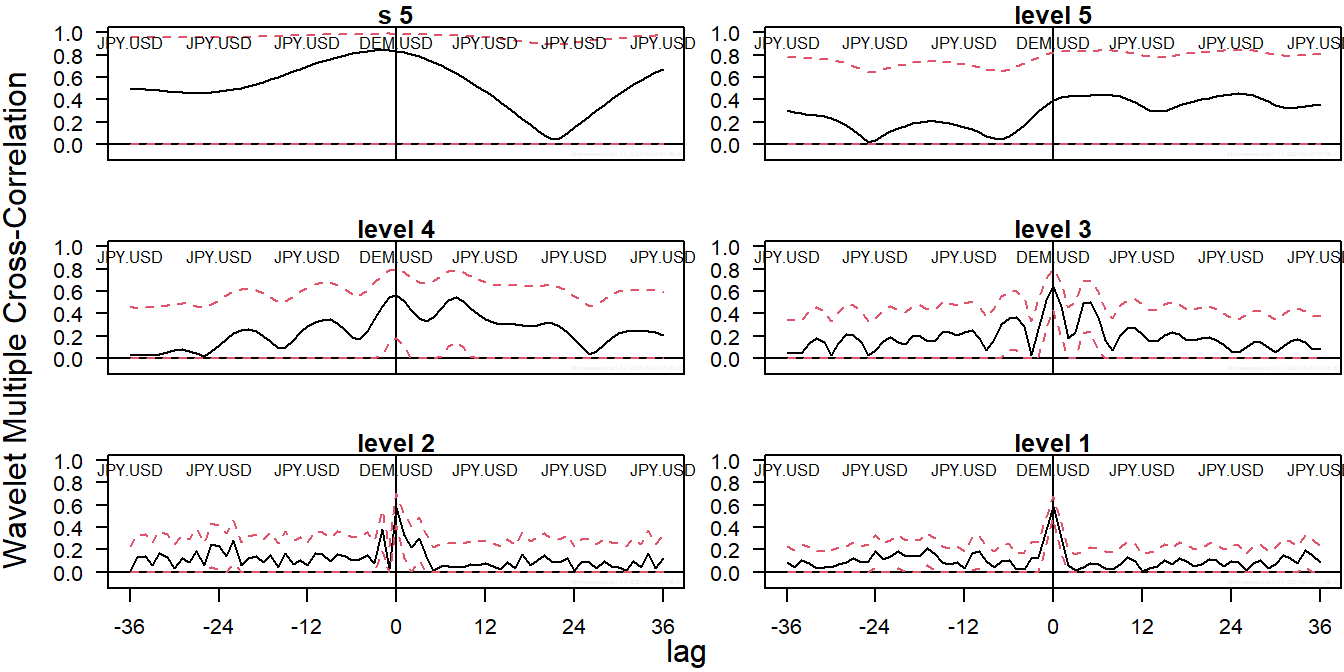true

## Introduction

Data evolution along time may be the consequence of heterogeneous causes occurring at different time scales or frequencies. This is not easily taken into account by traditional time series analysis (TSA) either in the time domain or in the frequency domain (Fourier analysis). These two approaches take opposite ways of exposing the information present in the time series under study. Thus, time domain TSA provides full time resolution at the cost of discarding all frequency information and, conversely, Fourier analysis has full frequency resolution but no information in time. The more recent wavelet analysis gets sort of in the middle by offering a compromise, with partial resolution in both time and frequency domains, so that we can separate periodic components at different timescales and observe how they evolve over time (Percival and Walden 2000; Aguiar-Conraria and Soares 2014).

### Wavelet correlation

Just like the standard correlation measure, wavelet correlation aims to evaluate the degree of association between two time series but on a scale-by-scale basis. Since the seminal work of Whitcher etal. (2000) and the release of the waveslim package (Whitcher 2015), pairwise wavelet correlations haven been widely used in many different scientific fields. However, standard wavelet correlations are restricted to pairs of time series. This is not helpful when dealing with a set of several time series since we would need to handle many wavelet correlation graphs and even more cross-correlation graphs. In this case, we would rather ask for a single measure of the overall association among them. Of course, this is even complicated further if we want to extend the comparison to the actual plethora of regression coefficients involved.

### Wavelet multiple regression and correlation

The wavelet multiple regression and correlation analysis proposed in Fernández-Macho (2012, 2018) extends wavelet correlation methodology to handle multivariate time series. As their names imply, the wavelet multiple regression (WMR) and cross-regression (WMCR) estimate a complete set of regression coefficients, together with their corresponding standard deviations and significance statistics, related to the overall statistical relationships that might exist at different timescales among observations on a multivariate time series. As special cases, the wavelet multiple correlation (WMC) and cross-correlation (WMCC) refer to just the actual measure of the degree of overall statistical relationships within the multivariate set of variables.

### Local vs. global wavelet multiple regression

Similarly, in a non-stationary context, the wavelet local multiple regression (WLMR) and correlation (WLMC) estimate and measure the time-evolving statistical relationships at different timescales within a multivariate data set. The usual practice of combining standard bivariate wavelet correlation analysis with rolling time windows is clearly inadequate as this needs to calculate, plot and compare a large number of wavelet regression and correlation graphs that now would require an additional time dimension. This renders pairwise multiscale comparisons pointless in practice. Besides, some consideration as to appropriateness of the usual rectangular rolling window needs to be taken into account. See Fernández-Macho (2018, 2019) for a brief introduction to wavelet methods and a discussion of the spectral properties of local windows needed for dynamic wavelet methods.

## The wavemulcor package

Package wavemulcor produces estimates of multiscale global or local multiple regressions and correlations along with approximate confidence intervals. It makes use of the maximal overlap discrete wavelet transform described in Percival and Walden (2000), and implemented by the modwt function in the waveslim R package developed by Whitcher (2015).

It contains several routines that calculate single sets of, respectively, global wavelet multiple regressions (WMC, WMR), global wavelet multiple cross-regressions (WMCC, WMCR) and time-localized wavelet multiple regressions (WLMC, WLMR) from a dataset of $$n$$ variables. The code is based on the calculation, at each wavelet scale $$\lambda_j$$, of the square root of the coefficient of determination in that linear combination of either global or locally weighted wavelet coefficients for which such coefficient of determination is a maximum. For reference, we can write such linear combination as $$w_{yj}=\beta w_{xj}$$, where $$w_{yj}$$ is the normalizing wavelet variable and $$\beta w_{xj}$$ is the linear combination of the rest of wavelet variables. Package wavemulcor can be obtained from The Comprehensive R Archive Network (CRAN) at https://cran.r-project.org/package=wavemulcor.

### WMR: wavelet multiple regression and correlation

These two wavelet routines produce estimates of multiscale multiple regressions together with their approximate confidence intervals.

The following example illustrates WMC with a tri-variate series consisting of the same exchange rate returns data as in Gençay etal. (2002), DEM-USD, JPY-USD, plus one random variable:

wf <- "d4"
J <- trunc(log2(N))-3
demusd.modwt <- brick.wall(modwt(returns[,"DEM.USD"], wf, J), wf)
jpyusd.modwt <- brick.wall(modwt(returns[,"JPY.USD"], wf, J), wf)
xrand.modwt <- brick.wall(modwt(rnorm(length(returns[,"DEM.USD"])), wf, J), wf)

xx <- list(demusd.modwt, jpyusd.modwt, xrand.modwt)
names(xx) <- c("DEM.USD","JPY.USD","rand")

Lst <- wave.multiple.correlation(xx)

The output from this function consists of a list of two elements:

• xy.mulcor, a $$J\times 3$$ matrix with as many rows as levels in the wavelet transform object. The first column provides the point estimate for the wavelet multiple correlation, followed by the lower and upper bounds from the confidence interval, and

• YmaxR, a numeric vector giving, at each wavelet level, the index number of the normalizing wavelet variable $$w_y$$ whose correlation is calculated (maximized by default) against a linear combination of the rest.

The output can be presented in a table or graph using the R code of our choice. A quick but nice option is to use the auxiliary routine plot_wave.multiple.correlation that produces a standard WMC plot:

plot_wave.multiple.correlation(Lst)The figure shows the wavelet multiple correlation for the exchange rate returns. The dashed lines correspond to the upper and lower bounds of the corresponding 95% confidence interval. The variables named on top1 give, at each scale, the largest multiple correlation obtained from a linear combination of the variables. As an option, we can overrule this by explicitly naming the normalizing variable.

For a complete regression output we can use WMR. A similar code chunk as the one before provides an example of its usage, but with the last line replaced by:

Lst <- wave.multiple.regression(xx)

It produces a first element with the same correlation output as wave.multiple.correlation plus a second element xy.mulreg with a list of relevant regression statistics, namely, the regression estimates at each wavelet level together with their standard deviations, confidence interval lower and upper bounds, the $$t$$ statistic values and p-values, and the indexes of the regressors when sorted by significance.

As before, this output can be fed into R code to produce tables and graphs of our choice. Alternatively, the auxiliary routine plot_wave.multiple.regression will give us a standard WMR plot:

plot_wave.multiple.regression(Lst) # nsig=2)The figure plots the wavelet multiple regression for the exchange rate returns, with different colors for each regression coefficient. The dashed lines correspond to the upper and lower bounds of their 95% confidence interval. Note that, in order not to crowd the plot excessively, we can choose to plot nsig regression coefficients only (the default is $$nsig=2$$.) As before, the variables named on top give, at each scale, the largest multiple correlation from a linear combination of the variables. The names of the nsig most significant regressors are also printed along the regression wavelet line. The shaded area represent the compound 95% significance interval around zero.

### WMCR: wavelet multiple cross-regression and cross-correlation

Wavelet cross-regressions are, in principle, the same as wavelet regressions but with a temporal time shift between the normalizing wavelet variable $$w_y$$ and its regressors $$w_x$$ at each of the wavelet levels. If the shift is positive (lag) we say that $$y$$ lags the rest of the variables at that wavelet level, if it is negative (lead) we say that $$y$$ leads.

The next example uses WMCR (or WMCC) with the same tri-variate series of exchange rate returns data as before:

wf <- "d4"
J <- trunc(log2(N))-3
lmax <- 36

demusd.modwt <- brick.wall(modwt(returns[,"DEM.USD"], wf, J), wf)
jpyusd.modwt <- brick.wall(modwt(returns[,"JPY.USD"], wf, J), wf)
rand.modwt   <- brick.wall(modwt(rnorm(length(returns[,"DEM.USD"])), wf, J), wf)

# ---------------------------

xx <- list(demusd.modwt, jpyusd.modwt, rand.modwt)
names(xx) <- c("DEM.USD","JPY.USD","rand")

Lst <- wave.multiple.cross.regression(xx, lmax)

The output from this function is made up of several elements:2

• xy.mulcor, similar as before but taking into account the time shifts. That is, it contains wavemulcor, lower, upper, three $$J\times lmax$$ matrices of correlation coefficients and corresponding lower and upper bounds confidence interval bounds.

• xy.mulreg, with a list of arrays containing relevant regression statistics for all wavelet levels, and lags and leads.

• YmaxR, with the same content as wave.multiple.regression before.

For a compact visualization with no confidence intervals, the cross-correlation output can be fed into a heatmap_ auxiliary routine to obtain a standard heat map representation the WMCC:

heatmap_wave.multiple.cross.correlation(Lst, lmax) #, by=3, ci=NULL, pdf.write=NULL)More generally, the output can also be fed into plot_ auxiliary routines to obtain a standard WMCC plot:

plot_wave.multiple.cross.correlation(Lst, lmax) #, by=2)…and a standard WMCR plot:

plot_wave.multiple.cross.regression(Lst, lmax) #, by=2)